Ex 3.5

Chapter 3 Class 10 Pair of Linear Equations in Two Variables (Term 1)
Serial order wise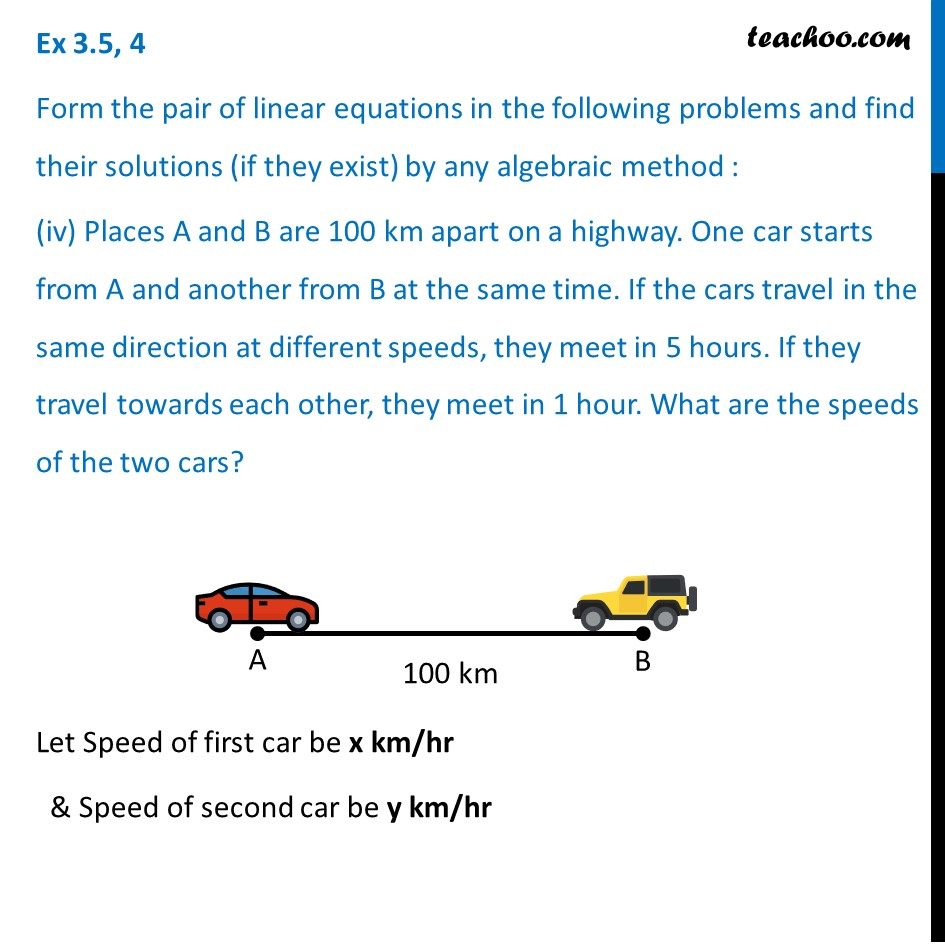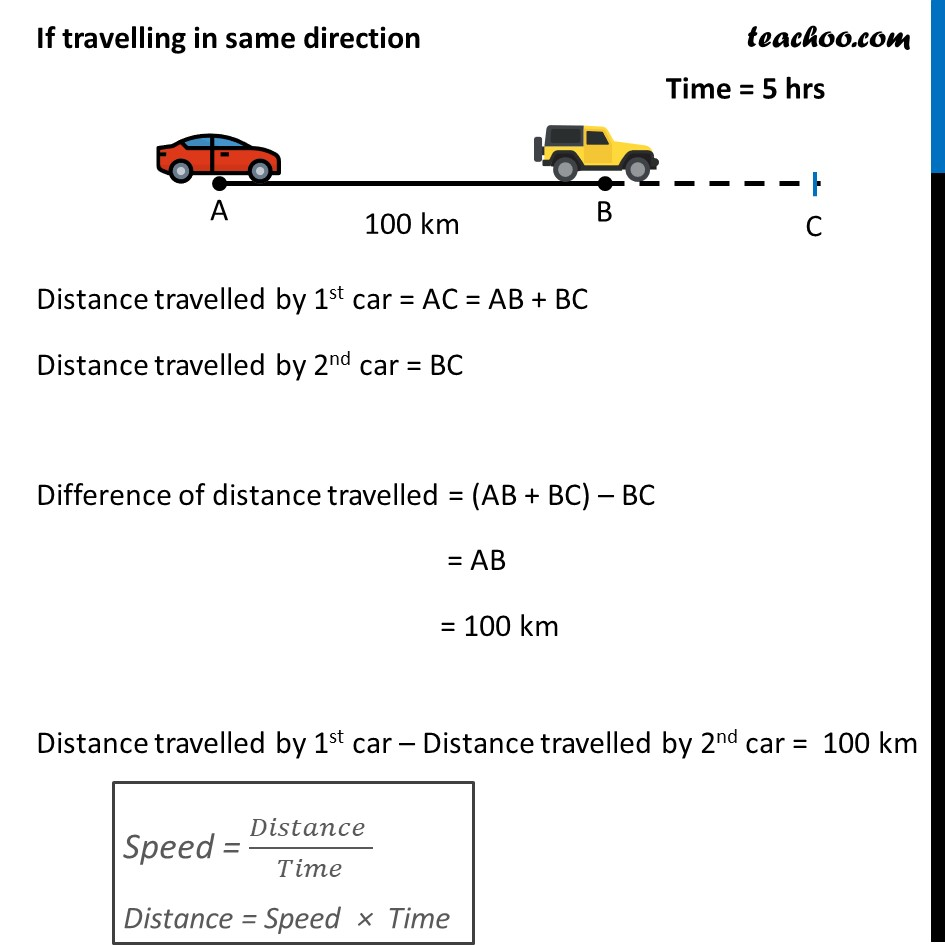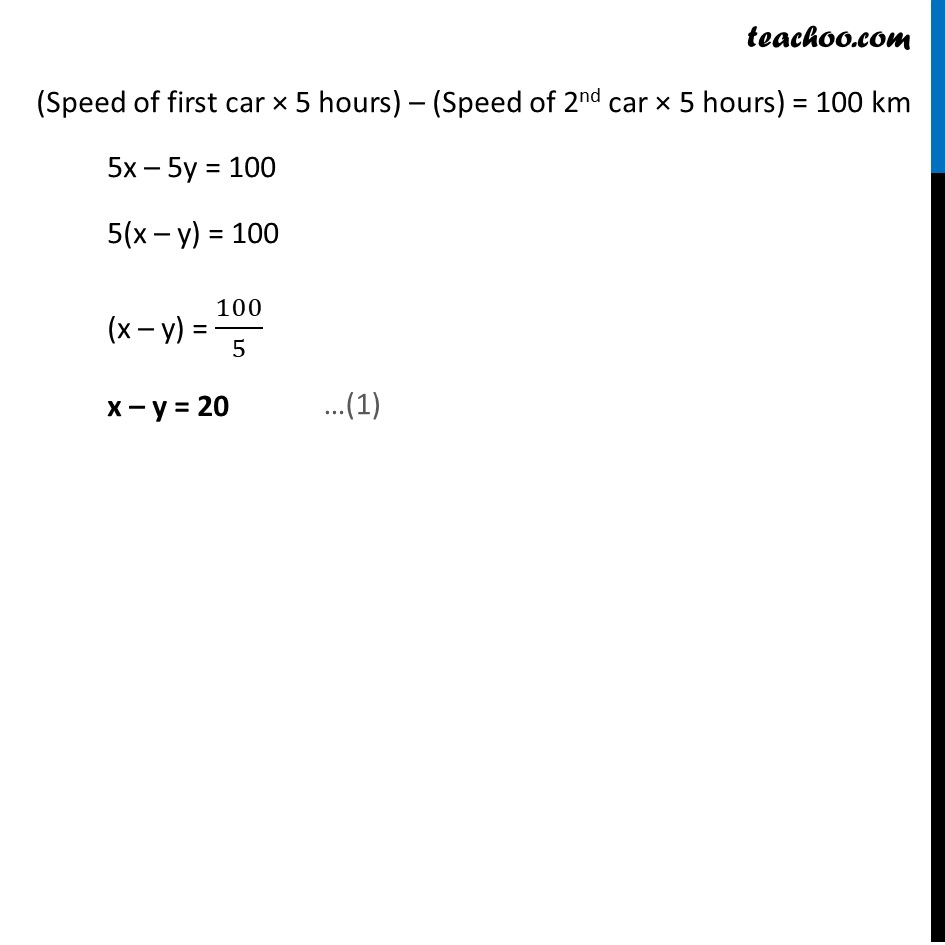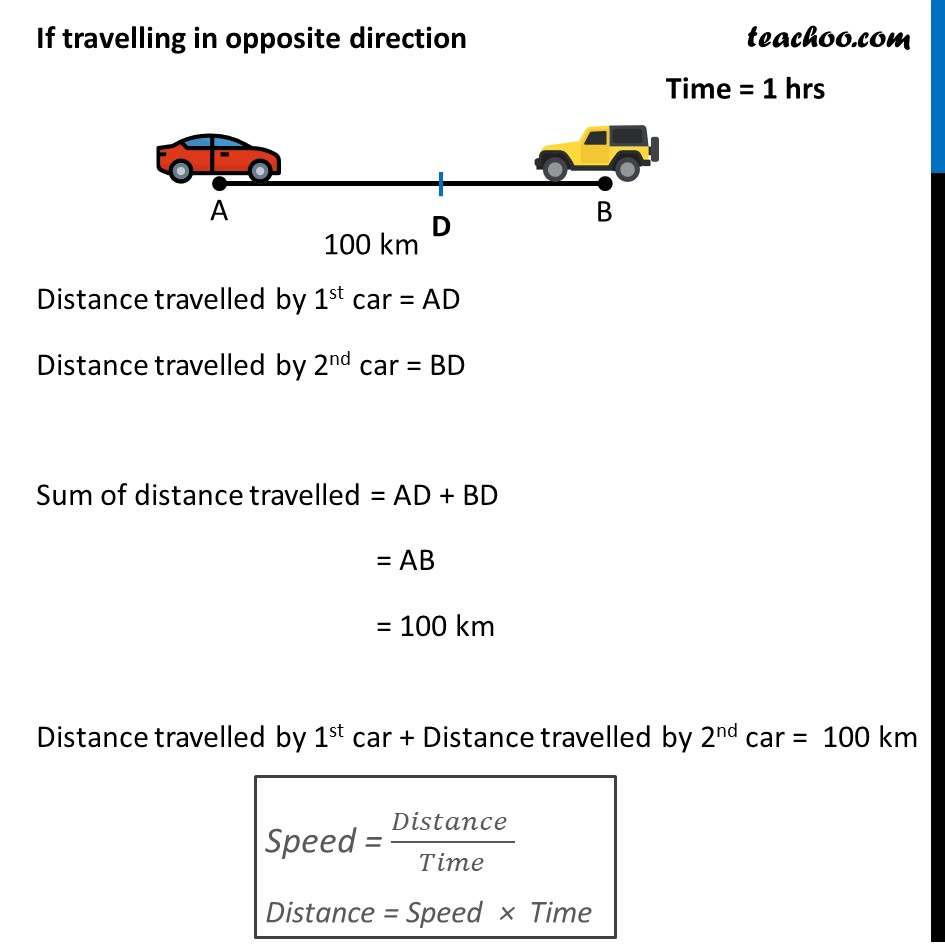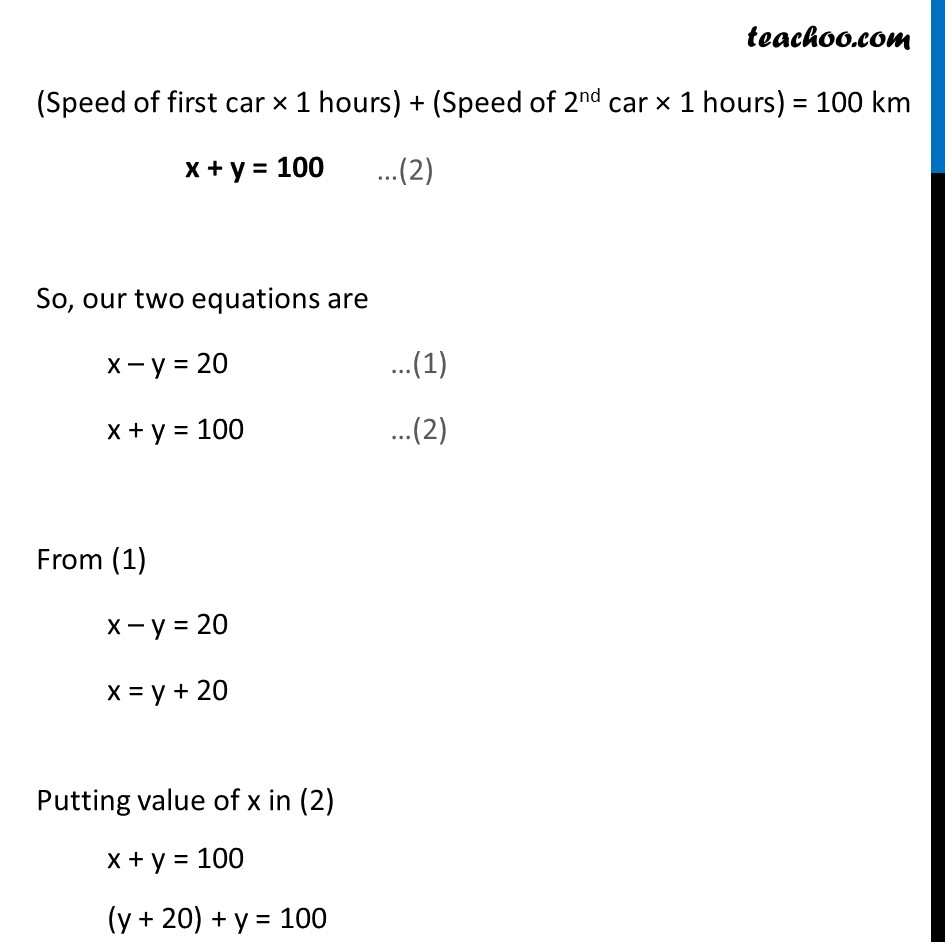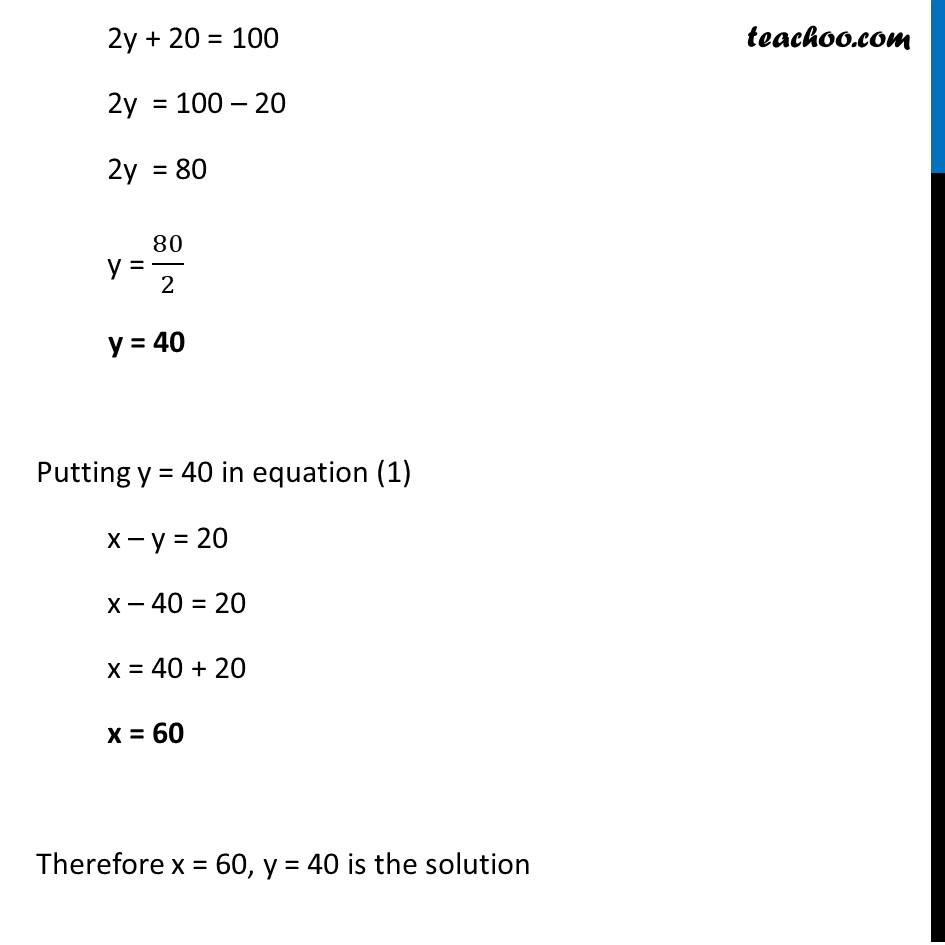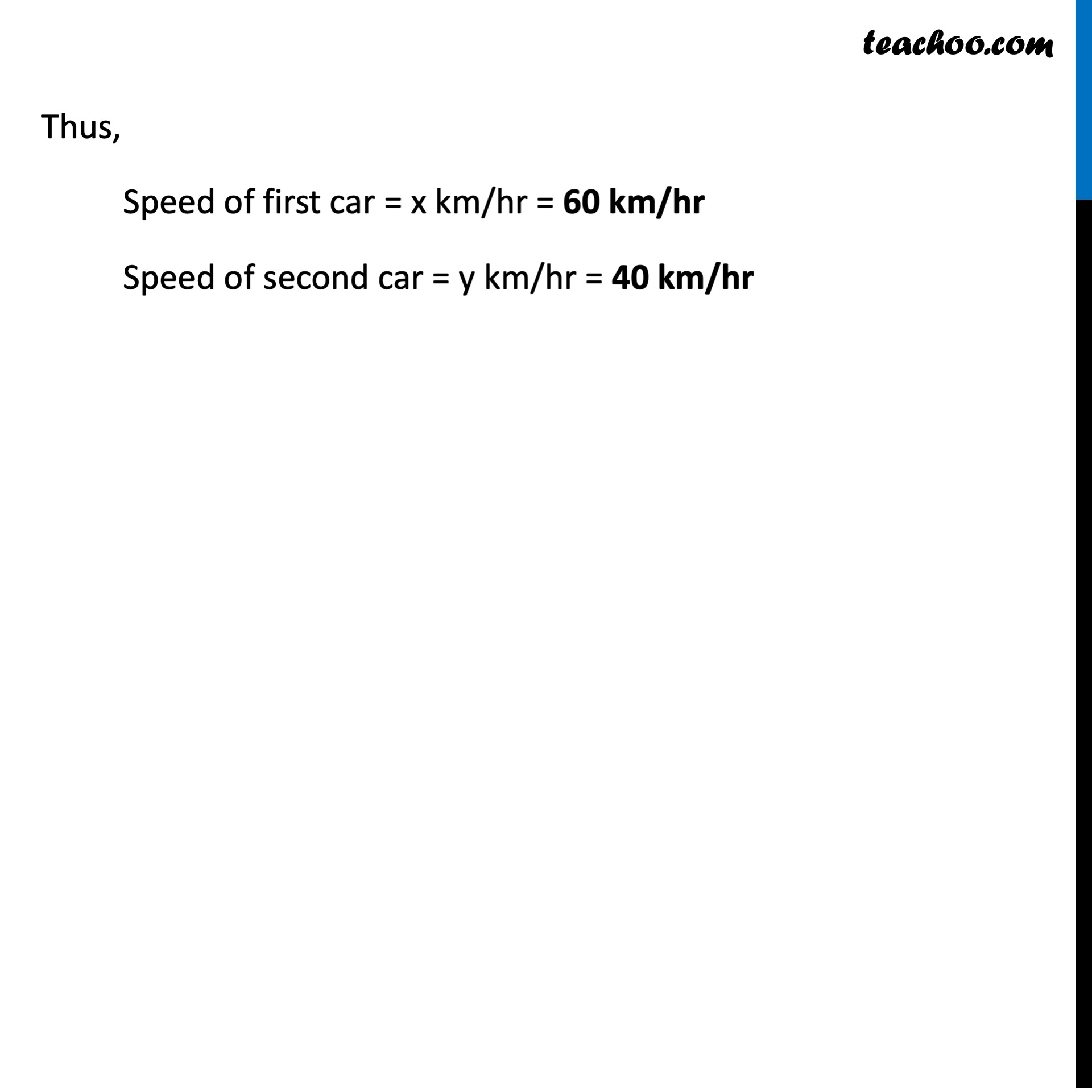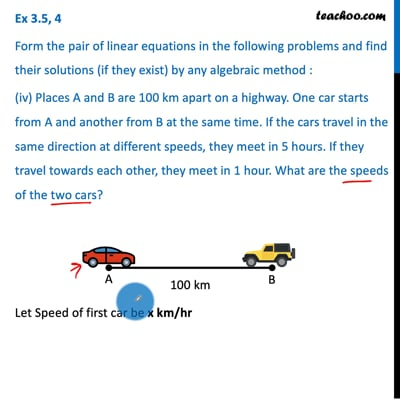This video is only available for Teachoo black users

### Transcript

Ex 3.5, 4 Form the pair of linear equations in the following problems and find their solutions (if they exist) by any algebraic method : (iv) Places A and B are 100 km apart on a highway. One car starts from A and another from B at the same time. If the cars travel in the same direction at different speeds, they meet in 5 hours. If they travel towards each other, they meet in 1 hour. What are the speeds of the two cars? Let Speed of first car be x km/hr & Speed of second car be y km/hr If travelling in same direction Distance travelled by 1st car = AC = AB + BC Distance travelled by 2nd car = BC Difference of distance travelled = (AB + BC) – BC = AB = 100 km Distance travelled by 1st car – Distance travelled by 2nd car = 100 km (Speed of first car × 5 hours) – (Speed of 2nd car × 5 hours) = 100 km 5x – 5y = 100 5(x – y) = 100 (x – y) = 100/5 x – y = 20 If travelling in opposite direction Distance travelled by 1st car = AD Distance travelled by 2nd car = BD Sum of distance travelled = AD + BD = AB = 100 km Distance travelled by 1st car + Distance travelled by 2nd car = 100 km (Speed of first car × 1 hours) + (Speed of 2nd car × 1 hours) = 100 km x + y = 100 So, our two equations are x – y = 20 …(1) x + y = 100 …(2) From (1) x – y = 20 x = y + 20 Putting value of x in (2) x + y = 100 (y + 20) + y = 100 2y + 20 = 100 2y = 100 – 20 2y = 80 y = 80/2 y = 40 Putting y = 40 in equation (1) x – y = 20 x – 40 = 20 x = 40 + 20 x = 60 Therefore x = 60, y = 40 is the solution Thus, Speed of first car = x km/hr = 60 km/hr Speed of second car = y km/hr = 20 km/hr